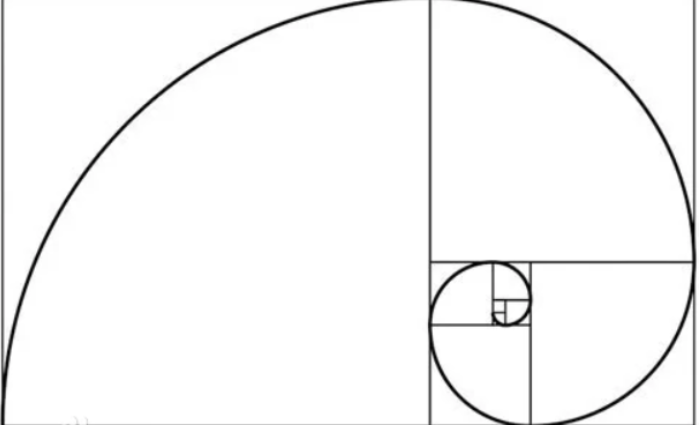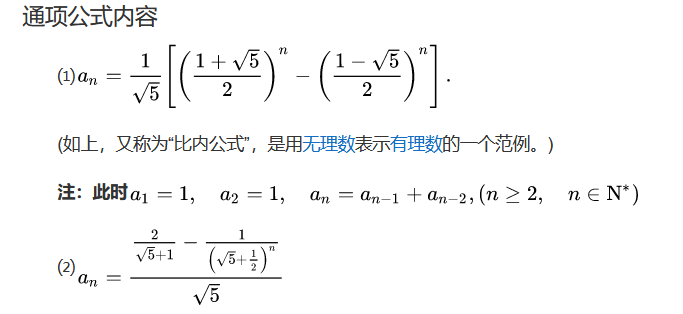# #打卡不停更#Python 之斐波那契数列的实现 原创

0收藏

## 1.斐波那契数列的概念

F(0)=0，

F(1)=1,

F(n)=

F(n - 1)+

F(n - 2)（

n ≥ 2，

n ∈ N*）在现代物理、准晶体结构、化学等领域，斐波那契数列都有直接的应用，为此，美国数学会从 1963 年起出版了以《斐波那契数列季刊》为名的一份数学杂志，用于专门刊载这方面的研究成果。## 3.解题方法

### （1）递归

``````def fib1(n):
if n == 1 or n == 2:
return 1
return fib1(n - 1) + fib1(n - 2)

for i in range(1, 21):
print(fib1(i), end=' ')``````

### （2）for循环

``````def fib2(n):
a, b = 0, 1
for i in range(n + 1):
a, b = b, a + b
return a

for i in range(20):
print(fib2(i), end=' ')``````帖子
视频
声望
粉丝
最近发布
热门推荐
相关问题
社区精华内容